•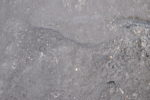•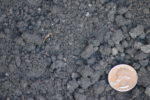•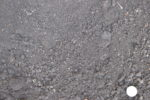•# Black Dirt #1 (Pulverized & Screened)

The blackest of black dirts. This premium black dirt is pulverized and screen. Perfect for landscaping, planting a backyard garden and filling in those pesky holes your dog keeps digging. It is also good to lay down prior to laying sod. Black dirt is one of the most versatile of our dirts.

• Pulverized and screen
• Great for gardens
• Our most versatile product
• Packaged in bags or by the yard
• Get in any quantity!
If you need help finding out your total cubic yardage use one of our calculators below!
Aerial Map Calculator
Area Totals
Current Area
0 ft2
Total Area
0 ft2
Total Measurement: 0 cubic yards
Total Price: \$0
Square Footage Calculator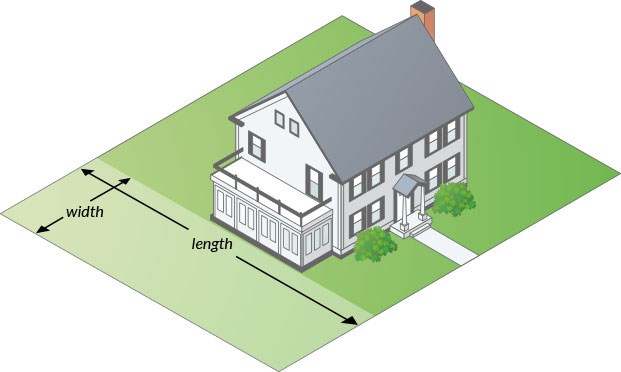• Area 1
• Area 1
• Area 1
Total Measurement: 0 cubic yards
Total Price: \$0

### How To Measure

#### Square or Rectangle

Measure the Length & Width and multiply.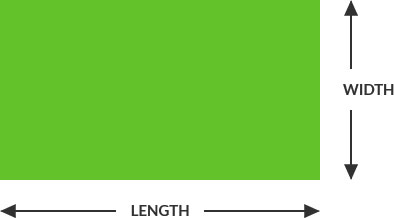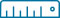Length x Width = Area
(12’ x 24’ = 288 ft2)

#### Right Triangle

Multiply the two sides that contact the right angle and divide by two.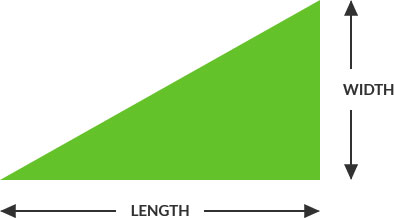Length x Width / 2 = Area
(12’ x 24’ = 288 ÷ 2 = 144 ft2)

#### Circular

Multiply the radius with the radius then multiply by 3.14. Radius is a straight line from the center of a circle to the outside of the circle.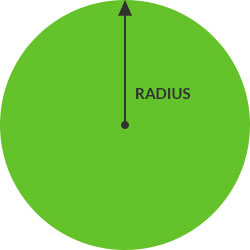##### Full Circle:(6’ x 6’ = 36 x 3.14 = 113 ft2)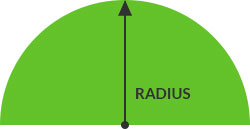##### Half Circle:Divide full circle radius by 2
(6’ x 6’ = 36 x 3.14 = 113 / 2 = 56.5 ft2)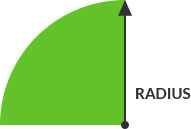##### Quarter Circle:Divide full circle radius by 4
(6’ x 6’ = 36 x 3.14 = 113 / 4 = 28.25 ft2)